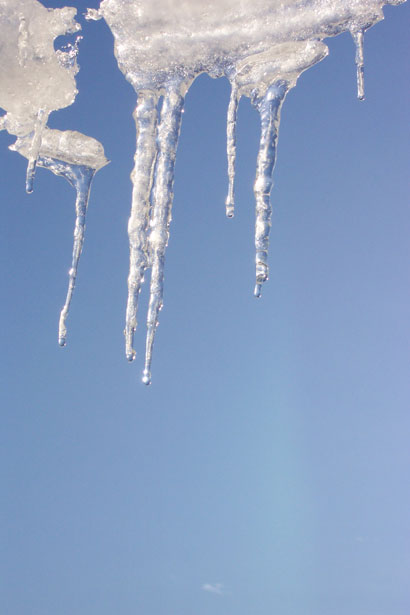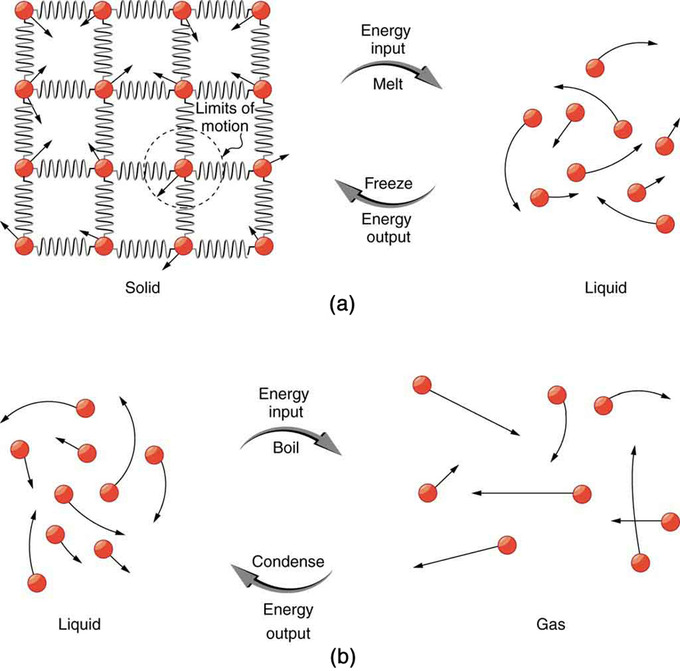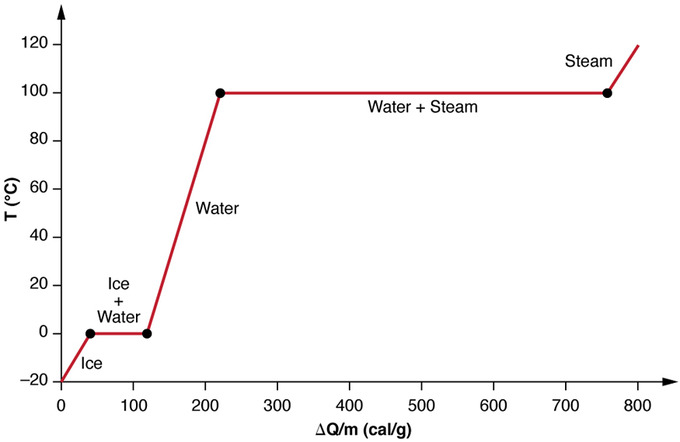## Latent Heat

The latent heat is the energy associated with a phase change of a substance.

### Learning Objectives

Describe the latent heat as a form of energy

### Key Takeaways

#### Key Points

• Energy is required to change the phase of a substance, such as the energy to break the bonds between molecules in a block of ice so it may melt.
• During a phase change energy my be added or subtracted from a system, but the temperature will not change. The temperature will change only when the phase change has completed.
• The heat Q required to change the phase of a sample of mass m is given by $\text{Q}=\text{mL}_{\text{f}}$ (melting or freezing) and $\text{Q}=\text{mL}_{\text{v}}$ (evaporating or condensing), where Lf and Lv are the latent heat of fusion and the latent heat of vaporization, respectively.

#### Key Terms

• latent heat of fusion: the energy required to transition one unit of a substance from solid to liquid; equivalently, the energy liberated when one unit of a substance transitions from liquid to solid.
• latent heat of vaporization: the energy required to transition one unit of a substance from liquid to vapor; equivalently, the energy liberated when one unit of a substance transitions from vapor to liquid.
• sublimation: the transition of a substance from the solid phase directly to the vapor state such that it does not pass through the intermediate, liquid phase

### Latent Heat

Previously, we have discussed temperature change due to heat transfer. No temperature change occurs from heat transfer if ice melts and becomes liquid water (i.e., during a phase change). For example, consider water dripping from icicles melting on a roof warmed by the Sun. Conversely, water freezes in an ice tray cooled by lower-temperature surroundings.Melting Icicle: Heat from the air transfers to the ice causing it to melt.

Energy is required to melt a solid because the cohesive bonds between the molecules in the solid must be broken apart so that the molecules can move around at comparable kinetic energies; thus, there is no rise in temperature. Similarly, energy is needed to vaporize a liquid, because molecules in a liquid interact with each other via attractive forces. There is no temperature change until a phase change is complete. The temperature of a glass of lemonade initially at 0 ºC stays at 0 ºC until all the ice has melted. Conversely, energy is released during freezing and condensation, usually in the form of thermal energy. Work is done by cohesive forces when molecules are brought together. The corresponding energy must be given off (dissipated) to allow them to stay together.

The energy involved in a phase change depends on two major factors: the number and strength of bonds or force pairs. The number of bonds is proportional to the number of molecules and thus to the mass of the sample. The strength of forces depends on the type of molecules. The heat Q required to change the phase of a sample of mass m is given by

$\text{Q}=\text{mL}_{\text{f}}$ (melting or freezing)

$\text{Q}=\text{mL}_{\text{v}}$ (evaporating or condensing)

where the latent heat of fusion, Lf, and latent heat of vaporization, Lv, are material constants that are determined experimentally.Phase Transitions: (a) Energy is required to partially overcome the attractive forces between molecules in a solid to form a liquid. That same energy must be removed for freezing to take place. (b) Molecules are separated by large distances when going from liquid to vapor, requiring significant energy to overcome molecular attraction. The same energy must be removed for condensation to take place. There is no temperature change until a phase change is complete.

Latent heat is an intensive property measured in units of J/kg. Both Lf and Lv depend on the substance, particularly on the strength of its molecular forces as noted earlier. Lf and Lv are collectively called latent heat coefficients. They are latent, or hidden, because in phase changes, energy enters or leaves a system without causing a temperature change in the system; so, in effect, the energy is hidden. Note that melting and vaporization are endothermic processes in that they absorb or require energy, while freezing and condensation are exothermic process as they release energy.

Heating Up Ice: Andrew Vanden Heuvel explores latent heat while trying to cool down his soda.

Significant amounts of energy are involved in phase changes. Let us look, for example, at how much energy is needed to melt a kilogram of ice at 0º C to produce a kilogram of water at 0°C. Using the equation for a change in temperature and the value for water (334 kJ/kg), we find that Q=mLf=(1.0kg)(334kJ/kg)=334kJ is the energy to melt a kilogram of ice. This is a lot of energy as it represents the same amount of energy needed to raise the temperature of 1 kg of liquid water from 0ºC to 79.8ºC. Even more energy is required to vaporize water; it would take 2256 kJ to change 1 kg of liquid water at the normal boiling point (100ºC at atmospheric pressure) to steam (water vapor). This example shows that the energy for a phase change is enormous compared to energy associated with temperature changes without a phase change.

Phase changes can have an enormous stabilizing effect (see figure below). Consider adding heat at a constant rate to a sample of ice initially at -20 ºC. Initially the temperature of the ice rises linearly, absorbing heat at a constant rate of 0.50 cal/g⋅C until it reaches 0 ºC. Once at this temperature, the ice begins to melt until all the entire sample has melted, absorbing a total of 79.8 cal/g of heat. The temperature remains constant at 0 ºC during this phase change. Once all the ice has melted, the temperature of the liquid water rises, absorbing heat at a new constant rate of 1.00 cal/g⋅C (remember that specific heats are dependent on phase). At 100ºC, the water begins to boil and the temperature again remains constant until the water absorbs 539 cal/g of heat to complete this phase change. When all the liquid has become steam, the temperature rises again, absorbing heat at a rate of 0.482 cal/g⋅C.Heating and Phase Changes of Water: A graph of temperature versus energy added. The system is constructed so that no vapor evaporates while ice warms to become liquid water, and so that, when vaporization occurs, the vapor remains in of the system. The long stretches of constant temperature values at 0ºC and 100ºC reflect the large latent heat of melting and vaporization, respectively.

A phase change we have neglected to mention thus far is sublimation, the transition of solid directly into vapor. The opposite case, where vapor transitions directly into a solid, is called deposition. Sublimation has its own latent heat Ls and can be used in the same way as Lvand Lf.